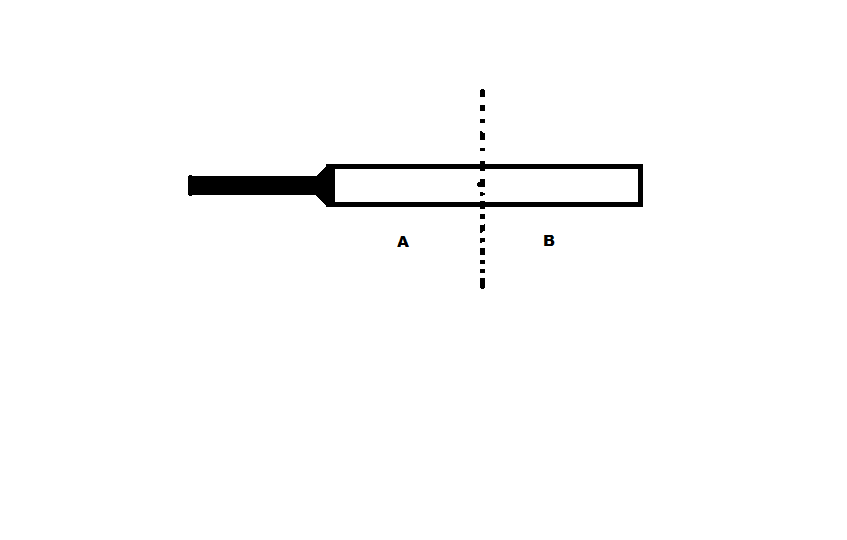# Some Physics To Do

There is a Cricket Bat which is cut from its center of mass as shown in figure.Then Which of the Following is True?

1) Mass of portion A = Mass of portion B

2) Mass of portion A > Mass of portion B

3) Mass of portion A < Mass of portion B

1, 2 or 3

×